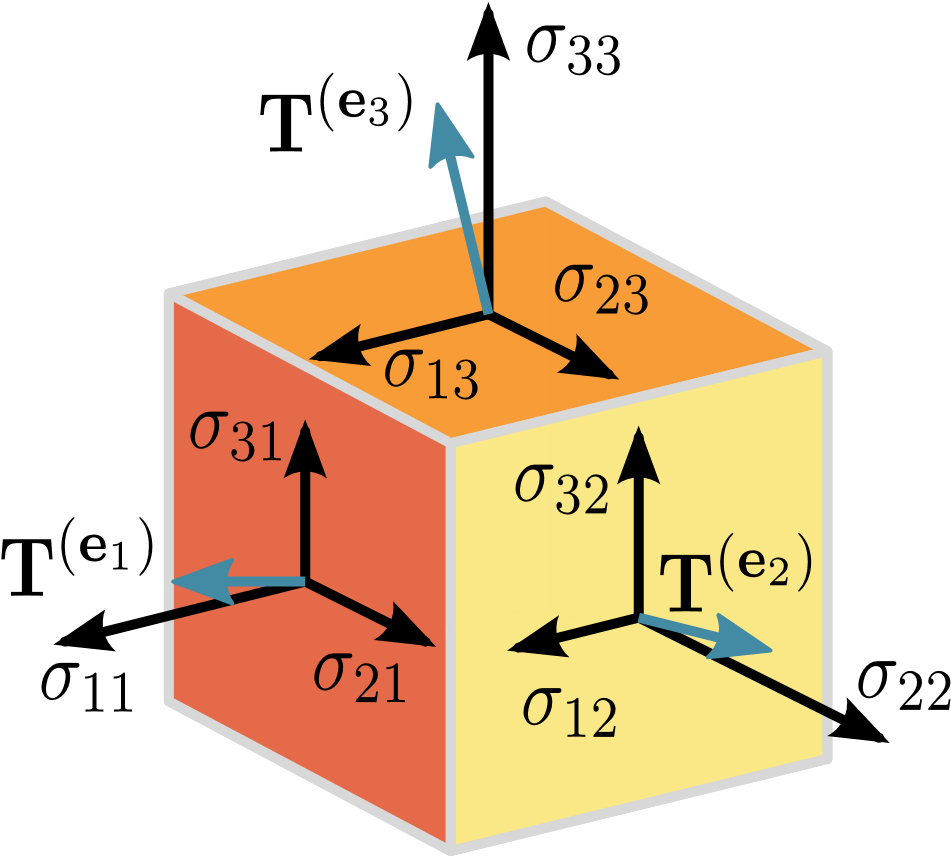# 在TensorFlow中怎么打印Tensor对象的值

``````matrix1 = tf.constant([[3., 3.]])
matrix2 = tf.constant([[2.],[2.]])
product = tf.matmul(matrix1, matrix2)
``````

``````<tensorflow.python.framework.ops.Tensor object at 0x10470fcd0>
``````

``````print product
Tensor("MatMul:0", shape=TensorShape([Dimension(1), Dimension(1)]), dtype=float32)
``````

Tensorflow编程指南中提到, 要在`Sessions`上运行的计算图，之后才能获取值。那么为了方便起见，有没有什么方法可以直接查看TensorObject的输出，而无需在`session`中运行计算图？

## 2.输出张量值的完整例子

``````# Initialize session
import tensorflow as tf
sess = tf.InteractiveSession()

# Some tensor we want to print the value of
a = tf.constant([1.0, 3.0])

# Add print operation
a = tf.Print(a, [a], message="This is a: ")

# Add more elements of the graph using a
b = tf.add(a, a).eval()
``````

``````I tensorflow/core/kernels/logging_ops.cc:79] This is a: [1 3]
``````

## 3.另外一个示例

``````import tensorflow as tf

#define a variable to hold normal random values
normal_rv = tf.Variable( tf.truncated_normal([2,3],stddev = 0.1))

#initialize the variable
init_op = tf.initialize_all_variables()

#run the graph
with tf.Session() as sess:
sess.run(init_op) #execute init_op
#print the random values that we sample
print (sess.run(normal_rv))
``````

``````[[-0.16702934  0.07173464 -0.04512421]
[-0.02265321  0.06509651 -0.01419079]]
``````

## 4.上述问题的可用完整代码

``````import tensorflow as tf
#Initialize the session
sess = tf.InteractiveSession()

matrix1 = tf.constant([[3., 3.]])
matrix2 = tf.constant([[2.],[2.]])
product = tf.matmul(matrix1, matrix2)

#print the product
print(product.eval())

#close the session to release resources
sess.close()
``````

## 5.另外一个极简的示例

``````import tensorflow as tf
sess = tf.InteractiveSession() # see the answers above :)
x = [[1.,2.,1.],[1.,1.,1.]]    # a 2D matrix as input to softmax
y = tf.nn.softmax(x)           # this is the softmax function
# you can have anything you like here
u = y.eval()
print(u)
``````

## 6.针对上面问题的分析及相应代码

• 构建计算图。
• 运行计算图。

``````matrix1 = tf.constant([[3., 3.]])
matrix2 = tf.constant([[2.],[2.]])
product = tf.matmul(matrix1, matrix2)
``````

``````init = tf.global_variables_initializer()
``````

``````sess = tf.Session()
// run variables initializer
sess.run(init)

print(sess.run([product]))
``````